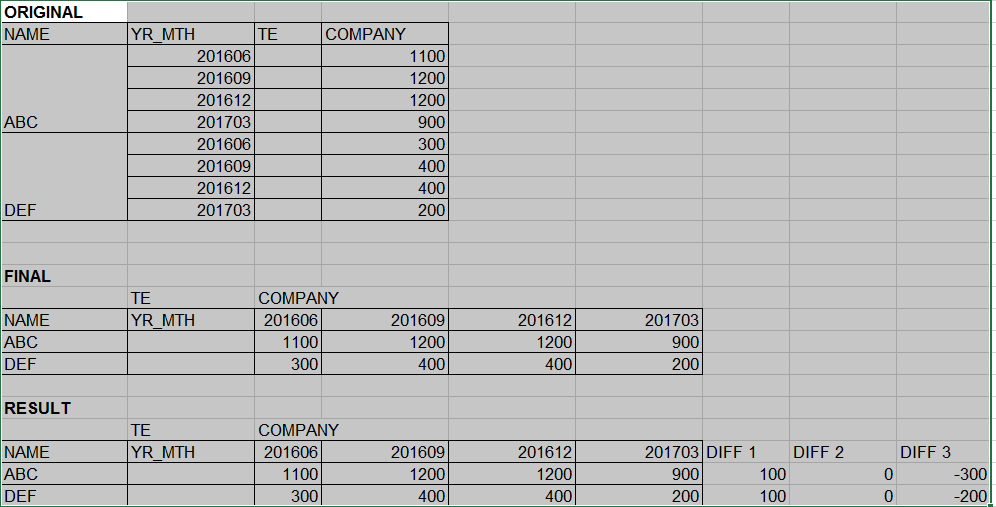Announcements
MAINTENANCE ALERT: Dec. 12th starting 9 AM CET. Community will be read-only. GET DETAILS
cancel
Showing results for
Did you mean:Creator II

## Dynamic 4 rolling quarter

I have a Date1 column in this format - YYYYMM.

Date1 = 201703 is 1st quarter

Date1 = 201706 is 2nd quarter

Date1 = 201709 is 3rd quarter

Date1 = 201712 is 4th quarter

Date1 = 201803 is 1st quarter

Date1 = 201806 is 2nd quarter

Date1 = 201809 is 3rd quarter

Date1 = 201812 is 4th quarter

How to create a rolling 4 quarter so that in a pivot table, the 4 quarters (as 4 columns) dynamically get updated?

1 Solution

Accepted SolutionsSpecialist

Hi,

='Q' & Right(Date1,2)/3

6 RepliesMVP

Are you looking to show Max 4 quarters in your pivot table? May be this

If Date1 is in number format.....

{<Date1 = {"\$(='>' & (Max(Date1)-100) & '<=' & Max(Date1))"}>}

If Date1 is in Datef format (like you mentioned)

{<Date1 = {"\$(='>' & Date(AddYears(Max(Date1), -1), 'YYYYMM') & '<=' & Date(Max(Date1), 'YYYYMM'))"}>}Specialist

Hi,

='Q' & Right(Date1,2)/3Creator II
Author

Hi Sunny, Yes I'm looking for last 4 quarters. I've the Date as a dimension in my pivot table, not as expression. How does this fit in this case?

Dimension is:
PICK(Dim, Date1, 'DIFF1', 'DIFF2', 'DIFF3')

Expression is:

Pick(Dim,
'\$'&Num(round(Sum(COMPANY) * 1.05),'###########,###'),
Num((Sum({<YR_MTH = {
"\$(=Min(YR_MTH, 2))"}>} COMPANY) - Sum({<YR_MTH = {"\$(=Min(YR_MTH, 1))"}>} COMPANY))/100, '#.0%'),
Num((Sum({<YR_MTH = {
"\$(=Min(YR_MTH, 3))"}>} COMPANY) - Sum({<YR_MTH = {"\$(=Min(YR_MTH, 2))"}>} COMPANY))/100, '#.0%'),
Num((Sum({<YR_MTH = {
"\$(=Min(YR_MTH, 4))"}>} COMPANY) - Sum({<YR_MTH = {"\$(=Min(YR_MTH, 3))"}>} COMPANY))/100, '#.0%'))Creator II
Author

Hi Fer Fer, that gave me 3 quarters from last year but dropped the current quarter. So, for instance if 201703 is the latest date which is 1st quarter 2017, I got Q2, Q3, Q4 for 2016 only and it dropped the 201703 (Q1 for 2017).Creator II
Author

Never mind, the Q1 didn't get dropped. It moved to the last column, so it wasn't in the same sorting order.Creator II
AuthorCommunity Browser